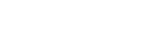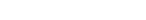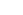# How to Make a Numpy Softmax Function

In this tutorial, I’ll show you how to define a Numpy softmax function in Python.

I’ll explain what the softmax function is. And I’ll show you the syntax for how to define the softmax function using the Numpy package.

Additionally, I’ll show you a few examples of how it works.

If you need something specific, you can click on any of the following links.

## Introduction to the Softmax Function

The softmax function is an s-shaped function that’s defined as:

(1)Typically, the input to this function is a vector of K real numbers. As an output, it produces a new vector of K real numbers that sum to 1. The values in the output can therefore be interpreted as probabilities that are related to the original input values.You’ll notice as well that as the softmax function “squishes” the input numbers into a range between 0 and 1, it tends to push the largest values closer to 1, and it pushes most of the other numbers towards 0.

Effectively, the softmax function identifies the largest value of the input. The largest “probability” in the output corresponds to the largest value of the input vector. In this sense, it is very similar to the argmax function. Softmax is like a continuous and differentiable version of argmax.

### Softmax is Commonly Used in Machine Learning

In data science, the softmax function is used most commonly in machine learning classification problems. In these use cases, the machine learning model typically produces a vector of real valued “scores” associated with each class, and the softmax function converts that vector of numbers into a vector of normalized numbers that can be interpreted as probabilities. In such a case, the “probabilities” generated by softmax are the probabilities associated with a particular class.

Because it’s commonly used in machine learning and deep learning, it’s potentially useful to know how to define the Softmax function “by hand.”

That being the case, let’s create a “Numpy softmax” function: a softmax function built in Python using the Numpy package.

## The syntax for a Python softmax function

Here, I’ll show you the syntax to create a softmax function in Python with Numpy.

I’ll actually show you two versions:

The reason for the “numerically stable” version is that the “basic” version can experience computation errors if we use it with large numbers. So, I’ll show you the syntax for the basic version, and then I’ll show you a corrected version.  We’ll look at examples of both later on in the examples section.

### Syntax: basic softmax

We can define a simple softmax function in Python as follows:

def softmax(x):
return(np.exp(x)/np.exp(x).sum())


#### A quick explanation of the syntax

Let’s quickly review what’s going on here.

Obviously, the def keyword indicates that we’re defining a new function. The name of the function is “softmax“.

The return() statement is returning a computation.

Here, using Numpy exponential and Numpy sum to compute the softmax function:

(2)Note also that I’ve used the Numpy sum method instead of the function, in order to enhance readability.  We could use the functional version, but the syntax would be slightly different.

### Syntax: Numerically Stable Softmax

Now, let’s define a slightly modified version.

The Numpy softmax function defined in the previous section actually has some problems. If the values in the input array are too large, then the softmax calculation can become “numerically unstable.”

This is because we’re computing the exponential of the elements of the input array. If those elements are very large, then we’re computing, and then using those numbers in a division calculation. No bueno.

To get around this, we can actually modify the code slightly, by subtracting the maximum value in the array from every value of the array. We can do this with the Numpy max function as follows:

def softmax_stable(x):
return(np.exp(x - np.max(x)) / np.exp(x - np.max(x)).sum())


Also, keep in mind that the structure of the inputs and outputs is essentially the same as the code for our “basic” softmax function seen above.

But as you’ll see in the examples, it works better when we have large numbers in the input array.

#### Format of the input values

Let’s briefly discuss the possible input values of the functions (this section will apply to both versions of our Numpy softmax function).

The softmax functions will operate on:

• single numbers
• Numpy arrays
• array-like objects (such as Python lists)

#### The output of the function

The output of this Numpy softmax function will be an array with the same shape as the input array.

But, the contents of the output array will be numbers between 0 and 1.

## Examples of Numpy Softmax

Now that we’ve looked at the syntax to define a Numpy softmax function, let’s look at some examples.

Examples:

#### Preliminary code: Import Numpy and Set Up Plotly

Before you run these examples, you need to run some setup code.

Specifically, you need to import Numpy and create some arrays we can operate on.

#### Import Packages

First, we need to import Numpy:

import numpy as np


Remember: we’re going to use np.exp to implement our Numpy softmax function.

#### Create Arrays

Next, we’ll create some input arrays that we can operate on.

Specifically, we’ll create an array with relatively “small” values, and we’ll also create an array with relatively “large” values (you’ll see why in a moment).

array_small = np.array([2, 11, 7])
array_large = np.array([555, 999, 111])


Now that we have our packages imported and we have some Numpy arrays, let’s run some examples.

### EXAMPLE 1: Use softmax on array with small values

First, we’ll use our basic softmax function on an array with small values.

softmax(array_small)


OUT:

array([1.21175444e-04, 9.81894794e-01, 1.79840305e-02])


#### Explanation

The values of array_small are [2, 11, 7].

But the output values are numbers between 0 and 1.

We can actually see them better if we round them:

softmax(array_small).round(2)


OUT:

array([0.  , 0.98, 0.02])


So the softmax function is taking the input values and squashing them into the range between 0 and 1. (We can actually interpret these like probabilities.)

Notice also that the output is still structured as a Numpy array, with the same shape as the input.

### EXAMPLE 2: Use Numpy softmax function on array with large values

Next, let’s use the basic softmax function on an array that contains large numbers.

Here, we’ll operate on array_large, which contains the values [555, 999, 111].

softmax(array_large)


OUT:

array([ 0., nan,  0.])


#### Explanation

As you can see, one of the values is nan. This indicates that there was a problem with the computation.

As explained earlier, the issue is that the computed exponents,are too large, which in turn, causes problems with the division that’s performed in the softmax computation.

### EXAMPLE 3: Use “numerically stable” Numpy softmax function on array with large values

Next, let’s use our “numerically stable” version of softmax.

In the previous example, we saw that the basic softmax function fails if we give it input values that are too large.

So here, we’ll use our numerically stable softmax function that we defined earlier.

(We’ll also apply the round() method, to make the output more readable.)

softmax_stable(array_large).round(2)


OUT:

array([0., 1., 0.])


#### Explanation

Here, we’ve used our softmax_stable() function to operate on  array_large

The input values inside array_large are [555, 999, 111].

When we use those values as the input to softmax_stable, the output values are [0., 1., 0.].

Essentially, this softmax output tells us that 999 is the largest number in the input values.

### EXAMPLE 4: Plot the softmax function

Finally, let’s plot what softmax looks like for a range of input values.

Specifically, we’ll use the numbers from 0 to 10 as the inputs.

#### Create x and y arrays

First, let’s just create the x and y values.

To create the x input values, we’ll use Numpy linspace to create an array of numbers from 0 to 10.

Then we’ll use the softmax() function to create the values that we’ll plot on the y-axis.

x_inputs = np.linspace(start = 0, stop = 10, num = 11)
y_softmax = softmax(x_inputs)


#### Plot

Now, we’ll plot.

Here, we’ll use the Plotly line function to create a line chart (although, you can also use the Seaborn lineplot function, which I’ve commented out).

px.line(x = x_inputs, y = y_softmax)
#sns.lineplot(x = x_inputs, y = y_softmax)


OUT:#### Discussion

You can see in the plot that the largest value of the input (10) corresponds to the largest y-value, which here is about .63.

What this shows us is that softmax has assigned the largest probability (.63) to the largest input value.

Additionally, it has assigned relatively small probabilities to most of the other input values. In particular, most of the relatively low input values correspond to output probabilities very close to 0.

As mentioned earlier: softmax tends to squish the relatively low input values towards 0, and pushes the higher values towards 1.

Do you have other questions about how to create or use a softmax function in Python?

In this tutorial, I’ve explained how implement and use a Numpy softmax in Python.

If you’re serious about mastering Numpy, and serious about data science in Python, you should consider joining our premium course called Numpy Mastery.

Numpy Mastery will teach you everything you need to know about Numpy, including:

• How to create Numpy arrays
• How to reshape, split, and combine your Numpy arrays
• What the “Numpy random seed” function does
• How to use the Numpy random functions
• How to perform mathematical operations on Numpy arrays
• and more …

Moreover, this course will show you a practice system that will help you master the syntax within a few weeks. We’ll show you a practice system that will enable you to memorize all of the Numpy syntax you learn. If you have trouble remembering Numpy syntax, this is the course you’ve been looking for.

Find out more here:

Joshua Ebner

Joshua Ebner is the founder, CEO, and Chief Data Scientist of Sharp Sight. Prior to founding the company, Josh worked as a Data Scientist at Apple. He has a degree in Physics from Cornell University.

### 1 thought on “How to Make a Numpy Softmax Function”

1. I wish I can have the same sort of material and explanation for the machine learning course in python which I am not able to see anywhere else.